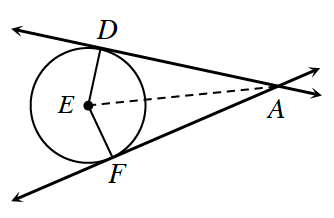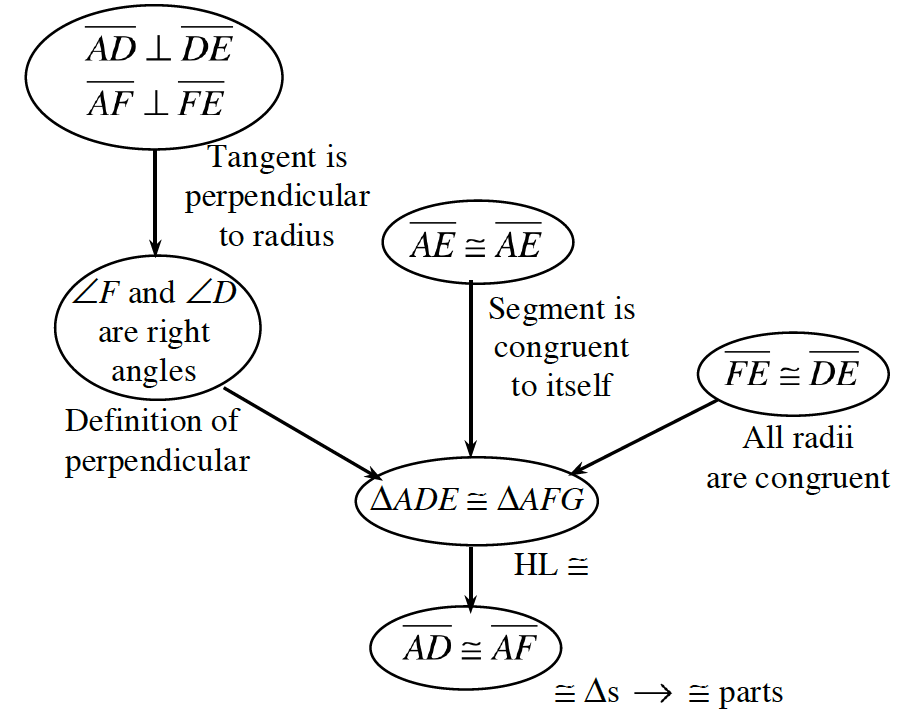### Home > INT2 > Chapter 11 > Lesson 11.2.2 > Problem11-72

11-72.Prove that when two lines that are tangent to a circle intersect, the distances from the point of intersection to the points of tangency are equal. That is, in the diagram at right, when $\overleftrightarrow { A D }$ is tangent to $⊙E$ at point $D$, and $\overleftrightarrow { A F }$ is tangent to $⊙E$ at point $F$, prove that $AD = AF$. Use either a
flowchart or a two-column proof.